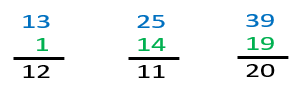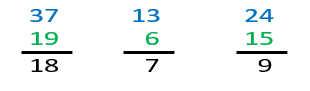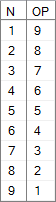# Virtual Indexing Part 7: Finite Opposite Numbers

Virtual Indexing continues…How do we to avoid sorting in either direction (ascending and descending)?## Introduction

This article is special for a few reasons, the first reason being that it’s about an idea that I came up with in Second grade and have built on since. This is arguably my favorite topic with respect to Virtual Indexing

## My “Opposite Numbers”

First for a few subtraction problems solved the way we did it in the 80’s:

Figure 1.1. Subtraction Series #1For me, these problems were very easy, each problem only requires one or two simple calculations. For the first problem (13-1) just subtract 1 from 3. For the next weI subtract 4 from 5, then 1 from 2 to get 11. I had subtraction down pat when subtracting smaller numbers from larger ones.  This next series would be more of a struggle:

Figure 1.2. Subtraction Series #2The first calculations for each problem involve subtracting a one-digit number from a two-digit number. For 37-19 the first step is to 9 from 17; for #2, the first step is to subtract 6 from 13; for the third it’s 5 from 14. For whatever reason I struggled with this so I came up with opposite numbers, not the ones you know but my own. Though I would not describe it this way then, the first step was to assign a relation between the numbers 1 through 9(N) and it’s “opposite”; it was a little auxiliary table in my brain. The opposite of 1 is 9 (O(1)=9) ,the opposite of 2 is 8 (O(2)=8), 3 is 7, 4 is 6, and 5 is 5. Note the table below:

Figure 2. Set of Numbers(N) and it’s “Opposite”Looking back at the problems in Figure 1.2 it was easier for me to first subtract 7 from 9 to get 2. The opposite of 2 is 8, then, each time I invoke my mental “opposite number” function I subtract 1 from the top number.  This was easier to me than remembering that 17-9=8. For the second problem (13-6) I calculate 6-3, then the opposite, which is 7. This can be expressed at O(6 – 3) = 7;

This made it possible for me to do subtraction much faster and is a mental technique I use to this day.

## Their Opposite Numbers

In 5th grade Pre Algebra we learned about Opposite Numbers (also known as additive inverse numbers.) Here the opposite of 5 is -5, the opposite of -20 is 20. Though okay with the concept, I was upset that someone took “Opposite Numbers” from me. Especially for something so trivial. In my head there were two types of opposite numbers: Mine and Theirs.

Their system of “opposite numbers” was important for understanding equations such as: (-(-9))-(5-(+5))-(-1). Then later for equations like: (-(-@A))-(@B-(+@B))-(-@C).  In the world of relational algebra, expressed using T-SQL we have:

``````DECLARE @A INT = 9, @B INT = 5, @C INT = 1;
SELECT (-(-@A))-(@B-(+@B))-(-@C); ``````

## Countable and Uncountable Infinity

This article could survive without this section but it’s relevant enough, and such a cool topic that I decided to slip it in. Did you know that there are different kinds of Infinity? For the purpose of this article let’s look at Countable Infinity. A set of Integers are countably infinite when “its elements can be put in one-to-one correspondence with the set of natural numbers. In other words, one can count off all elements in the set in such a way that, even though the counting will take forever.”

For example, this series in countably infinite because you can always add another number(1):
1,2,3,4,5,6,7

This sequence is as well:
0, -3, -6, -9, -13

Uncountable infinity looks more like:
0.1, 0.2, 0.3… 0.9, 0.11, 0.12…. 0.99999991, 0.99999992

Uncountable infinity demonstrates that there is an infinite amount of numbers between 0 and 1. I only included this so you can better understand what Countable Infinity is. Moving on…

## Introducing Finite Opposite Numbers

My opposite numbers” and “their opposite numbers” works in my head but, to pass the Idea I’m about to cover to others I had to come up with better nomenclature. What do my opposite numbers have in common with their opposite numbers? Each number in the set has an “opposite”, a value which is equal distance away from an arbitrary center point, AKA median. I say arbitrary because: who made 0 the official median value? Any number can represent a median right? 0 works for traditional opposite numbers because the set is infinite. Without an upper-bound or lower-bound values ; the numbers are countably infinite in two directions beginning with 0.

Figure 3.1. Infinite Sequence traveling away from 0

This infinite set of numbers with zero as the median is what I have coined, Infinite Opposite Numbers.  When we are dealing with a finite set with both an upper and lower bound, I came up with Finite Opposite Numbers. Let’s take the numbers 1 through 11. Here the median is 6; O(5) = 7, O(4) = 8, O(3) = 9, etc.

Figure X. Infinite Sequence traveling away from 6

Assigning upper and lower bounds moves the median value.

Figure 3.2. How the median shifts

In the example above we have an odd number of rows, what happens when the rowcount is even? Consider the difference between 1-7 and 1-6:

Figure 3.3. Infinite Sequence traveling away from 0

With the first example, 4 is the median, what is the median for the numbers 1-6?  “3.5” is not a valid integer.  For even numbers we’ll consider the two numbers closest to the middle (3.5 is in this example): the median would be the tuple containing the numbers 3 & 4 as a tuple in this example. To handle both odd and even sets I came up with this simple formula to calculate the opposite number: H+LN

## The Finite Opposites Function

This may seem unnecessary to create a function for this calculation but I use it so that others know what I’m doing, especially considering that this is a new concept. As a T-SQL function we have:

Figure 4: Finite Opposite Number Logic

``````CREATE FUNCTION [dbo].[O] (@L INT,@H INT,@N INT)
RETURNS TABLE WITH SCHEMABINDING AS RETURN SELECT Op = @L+@H-@N;``````

Here’s the entire function with comments.

### Basic Use

In each of these examples the function accepts the parameters L, H, and N then builds a set of numbers from L to H. Returning the finite opposite for each N in the set.

Figure 5. Basic usage examples

``````DECLARE @L INT = 1, @H INT = 5;

--==== 1. Run dbo.fnTally
SELECT
N  = f.N,
OP = N.Op
FROM        dbo.fnTally(@L,@H) AS f
CROSS APPLY o(@L,@H,f.N) AS N;

--==== 2. Set new values for @L and @H
SELECT @L = -2, @H = 2;

--==== Run dbo.GetNums
SELECT
N  = f.N,
OP = N.Op
FROM        dbo.GetNums(-2,2) AS f
CROSS APPLY o(@L,@H,f.N) AS N;``````

Figure 6. Basic example results

### Understanding the median tuple (1 or 2 members)

Below is an example of how the median value(s) are assigned.

Figure 7.1. Calculating the median

``````--==== 1. Even row count
DECLARE @L INT = 1, @H INT = 6;

SELECT
N  = f.N,  -- N
OP = N.Op, -- O(N)
DM = ABS(f.N-N.OP)/2 -- Distance from the median
FROM        dbo.fnTally(@L,@H) AS f
CROSS APPLY o(@L,@H,f.N) AS N;
GO
--==== 1. Even row count
DECLARE @L INT = 1, @H INT = 7;

SELECT
N  = f.N,  -- N
OP = N.Op, -- O(N)
DM = ABS(f.N-N.OP)/2 -- Distance from the median
FROM        dbo.fnTally(@L,@H) AS f
CROSS APPLY o(@L,@H,f.N) AS N;``````

7.2. Odd/Even row counts

## Quick Review

We have now defined types of “Opposite Numbers”:

1. Infinite opposite numbers (no upper or lower boundaries, 0 is the median)
2. Finite opposite numbers (both the upper and lower boundaries are defined, the median is calculated at ABS(N-OP)/2

## Examples

There are a couple examples of finite opposites here. Below are a couple basic examples.

### Example #1: A Home-Grown REVERSE Function

Let’s start with a fun string problem and create our own REVERSE function. We can start by tokenizing the string into unigrams. I’ll use the old FOR XML PATH trick and using STRING_AGG for those on 2017+.

Figure 8. Home-grown REVERSE examples

``````--==== 1. Basic REVERSE Function
DECLARE
@String     VARCHAR(8000) = 'ABC-123-XYZ',
@pattern    VARCHAR(100)  = '%[A-Z]%';

--== 1.1. Using STRING_AGG (SQL 2017+)
SELECT
Original = @String,
Reversed = STRING_AGG(SUBSTRING(@string,N.Op,1),'') WITHIN GROUP(ORDER BY f.N)
FROM        dbo.fnTally(1,LEN(@String)) AS f
CROSS APPLY dbo.o(1,LEN(@String),f.N)   AS N;

--== 1.2. Using FOR XML PATH (2005 to 2014)
SELECT
Original = @String,
Reversed = f.String
FROM
(
SELECT      SUBSTRING(@string,N.Op,1)
FROM        dbo.fnTally(1,LEN(@String)) AS f
CROSS APPLY dbo.o(1,LEN(@String),f.N)   AS N
ORDER BY f.N
FOR XML PATH('')
) AS f(String);``````

Each of these works as expected. The performance can’t match the T-SQL REVERSE, this is an example of how to return data in either direction leveraging the Virtual Index to avoid a sort. Now let’s say you need a function to return the first N number of characters that match a pattern and need the option to return the string in a user-defined direction (forward or backward).

Figure 9. Custom REVERSE Function Examples

``````--==== 2. Get first/Last @matches number of characters that match @pattern
DECLARE
@String     VARCHAR(8000) = 'ABC-123-XYZ',
@pattern    VARCHAR(100)  = '%[A-Z]%',
@matches    BIGINT        = 4,
@descending BIT           = 0;

SELECT STRING_AGG(f.chars,'')
FROM
(
SELECT TOP(@matches) chars = a.Txt
FROM        dbo.fnTally(1,LEN(@String)) AS f
CROSS APPLY dbo.o(1,LEN(@String),f.N)   AS N
CROSS APPLY (VALUES(IIF(@descending=0,
SUBSTRING(@string,f.N,1),
SUBSTRING(@string,N.Op,1)))) AS a(Txt)
WHERE       a.Txt LIKE @pattern
) AS f;``````

### Example #2: Sortless Parallel Apply

I’ll wrap up with this as it’s an excellent example of finite opposites in use. In Itizik Ben-Gan’s Microsoft SQL Server 2012 High-Performance T-SQL Using Window Functions Ben Gan demonstrates the “Parallel APPLY” trick, a way to improve the parallel plan produced by the optimizer. The code below is the setup code from the book (I misplace mine so I can’t correctly cite this, sorry.)

Figure 10. Parallel APPLY setup code (from the book above)

``````BEGIN
IF OBJECT_ID('dbo.Transactions','U') IS NOT NULL DROP TABLE dbo.Transactions;
IF OBJECT_ID('dbo.Accounts','U')     IS NOT NULL DROP TABLE dbo.Accounts;
CREATE TABLE dbo.Accounts
(
actid   INT         NOT NULL,
actname VARCHAR(50) NOT NULL,
CONSTRAINT PK_Accounts PRIMARY KEY(actid)
);
CREATE TABLE dbo.Transactions
(
actid  INT   NOT NULL,
tranid INT   NOT NULL,
val    MONEY NOT NULL,
CONSTRAINT PK_Transactions          PRIMARY KEY(actid, tranid),
CONSTRAINT FK_Transactions_Accounts FOREIGN KEY(actid) REFERENCES dbo.Accounts(actid)
);

INSERT INTO dbo.Accounts(actid, actname)
VALUES(1,'account 1'),(2,'account 2'),(3,'account 3');
INSERT INTO dbo.Transactions(actid, tranid, val) VALUES
(1, 3, 5.00),(1, 4, 2.00),(1, 5, 1.00),(1, 6, 3.00),(1, 7,-4.00),(1, 8,-1.00),
(1, 9,-2.00),(1,10,-3.00),(2, 1, 2.00),(2, 2, 1.00),(2, 3, 5.00),(2, 4, 1.00),
(2, 5,-5.00),(2, 6, 4.00),(2, 7, 2.00),(2, 8,-4.00),(2, 9,-5.00),(2,10, 4.00),
(3, 1,-3.00),(3, 2, 3.00),(3, 3,-2.00),(3, 4, 1.00),(3, 5, 4.00),(3, 6,-1.00),
(3, 7, 5.00),(3, 8, 3.00),(3, 9, 5.00),(3,10,-3.00),(1, 1, 4.00),(1, 2,-2.00);

CREATE INDEX idx_actid_val_i_tranid
ON dbo.Transactions(actid /* P */, val /* O */)
INCLUDE(tranid /* C */);
END;``````

Let’s compare the original Parallel APPLY technique then another which leverages my finite opposites function (dbo.O). For this to work I need the total number of rows as my upper-bound. I don’t have the row count ahead of time I can use COUNT as a window aggregate partitioned by actid.

Figure 11. Parallel APPLY with/without Finite Opposites

``````PRINT CHAR(10)+('Parallel APPLY')+CHAR(10)+REPLICATE('-',90);
SELECT
c.actid,
fn_pre.tranid,
fn_pre.val,
fn_pre.rownumasc,
fn_pre.rownumdesc
FROM  dbo.Accounts AS c
CROSS APPLY
(
SELECT t.tranid, t.val,
rownumasc  = ROW_NUMBER() OVER(ORDER BY t.val),
rownumdesc = ROW_NUMBER() OVER(ORDER BY t.val DESC)
FROM  dbo.Transactions AS t
WHERE t.actid = c.actid
) AS fn_pre
OPTION (QUERYTRACEON 8649);
GO

--== 1.6.4. Parallel APPLY + Finite Opposites
PRINT CHAR(10)+('Parallel APPLY+ Finite Opposites')+CHAR(10)+REPLICATE('-',90);
SELECT
actid      = c.actid,
tranid     = fn_pre.tranid,
val        = fn_pre.val,
rownumasc  = fn_pre.rownumasc,
rownumdesc = n.Op
FROM dbo.Accounts AS c
CROSS APPLY
(
SELECT t.tranid, t.val,
rownumasc = ROW_NUMBER() OVER(ORDER BY t.val),
rowz      = COUNT(*) OVER (PARTITION BY t.actid ORDER BY (SELECT NULL))
FROM   dbo.Transactions AS t
WHERE  T.actid = C.actid
) AS fn_pre
CROSS APPLY dbo.o(1,fn_pre.rowz-fn_pre.rownumasc+1,fn_pre.rownumasc) AS N
OPTION (QUERYTRACEON 8649);``````

Figure 12. Execution Plans

Note the second plan does not require a sort.

I will update this article with some performance test results later this week. I realized a small test error as I would about to publish this. That said, the sortless version is faster. I promise.

## Conclusion

Ordering rows in both Ascending and Descending order without a sort. Happy 2021!## Author: Alan Burstein

SQL Performance Ninja with 20+ years of writing high-performing, elegant SQL code. Expert at developing super fast NLP algorithms

Posted on Tags

## 2 thoughts on “Virtual Indexing Part 7: Finite Opposite Numbers”

1.Adam Machanic says:

Interesting idea. A few comments regarding your modification to the Parallel APPLY:
– First of all, note that the new query outputs different results for the descending sort. That may or may not be a problem, as the rows are properly sorted. But you should be able to produce identical output if you fix the math.
– Also, your window frame on the COUNT(*) is already implicitly partitioned by ‘actid’ because your APPLY is correlated by that column.
– Finally, you’ve eliminated the sort, but you’ve replaced it with a window spool. For small numbers of transactions per account this may be okay, but it doesn’t scale nearly as well. Use the code below to bloat the transactions table, turn off rendering of results in the grid, and check out the actual run time on both queries. Note that query cost is *always* an estimate, and often a very poor one.

insert into dbo.transactions
select actid, tranid + (number * 100), val
from dbo.transactions as t
cross join master..spt_values as v
where
v.type = ‘p’
and v.number > 0;

insert into dbo.transactions
select actid, tranid + (number * 1000000), val
from dbo.transactions as t
cross join master..spt_values as v
where
v.type = ‘p’
and v.number between 1 and 100;